## Agenda

• Toy collector solution
• Plug-In and the Bootstrap
• Nonparametric and Parametric Bootstraps
• Examples

## Exercise: Toy Collector

Children (and some adults) are frequently enticed to buy breakfast cereal in an effort to collect all the action figures. Assume there are 15 action figures and each cereal box contains exactly one with each figure being equally likely.

• Find the expected number of boxes needed to collect all 15 action figures.
• Find the standard deviation of the number of boxes needed to collect all 15 action figures.
• Now suppose we no longer have equal probabilities, instead let
Figure A B C D E F G H I J K L M N O
Probability .2 .1 .1 .1 .1 .1 .05 .05 .05 .05 .02 .02 .02 .02 .02
• Estimate the expected number of boxes needed to collect all 15 action figures.
• What is the uncertainty of your estimate?
• What is the probability you bought more than 300 boxes? 500 boxes? 800 boxes?

## Exercise: Toy Collector

• Consider the probability of the "new toy" given we already have $$i$$ toys
• Then $P(\text{New Toy} | i) = \frac{15-i}{15}$
• Then since each box is independent, our waiting time until a "new toy" is a geometric random variable
• The mean is $\frac{15}{15} + \frac{15}{14} + \dots + \frac{15}{1} \approx 49.77$
• The variance is $\frac{15(1-15/15)}{15} + \frac{15(1-14/15)}{14} + \dots + \frac{15(1-1/15)}{1} \approx 34.77$ with standard deviation 5.90

## Exercise: Toy Collector

prob.table <- c(.2, .1, .1, .1, .1, .1, .05, .05, .05, .05, .02, .02, .02, .02, .02)
boxes <- seq(1,15)
box.count <- function(prob=prob.table){
check <- double(length(prob))
i <- 0
while(sum(check)<length(prob)){
x <- sample(boxes, 1, prob=prob)
check[x] <- 1
i <- i+1
}
return(i)
}

## Exercise: Toy Collector

trials <- 1000
sim.boxes <- double(trials)
for(i in 1:trials){
sim.boxes[i] <- box.count()
}
est <- mean(sim.boxes)
mcse <- sd(sim.boxes) / sqrt(trials)
interval <- est + c(-1,1)*1.96*mcse
est
##  115.468
interval
##  112.0715 118.8645

## Exercise: Toy Collector

hist(sim.boxes, main="Histogram of Total Boxes", xlab="Boxes")
abline(v=300, col="red", lwd=2)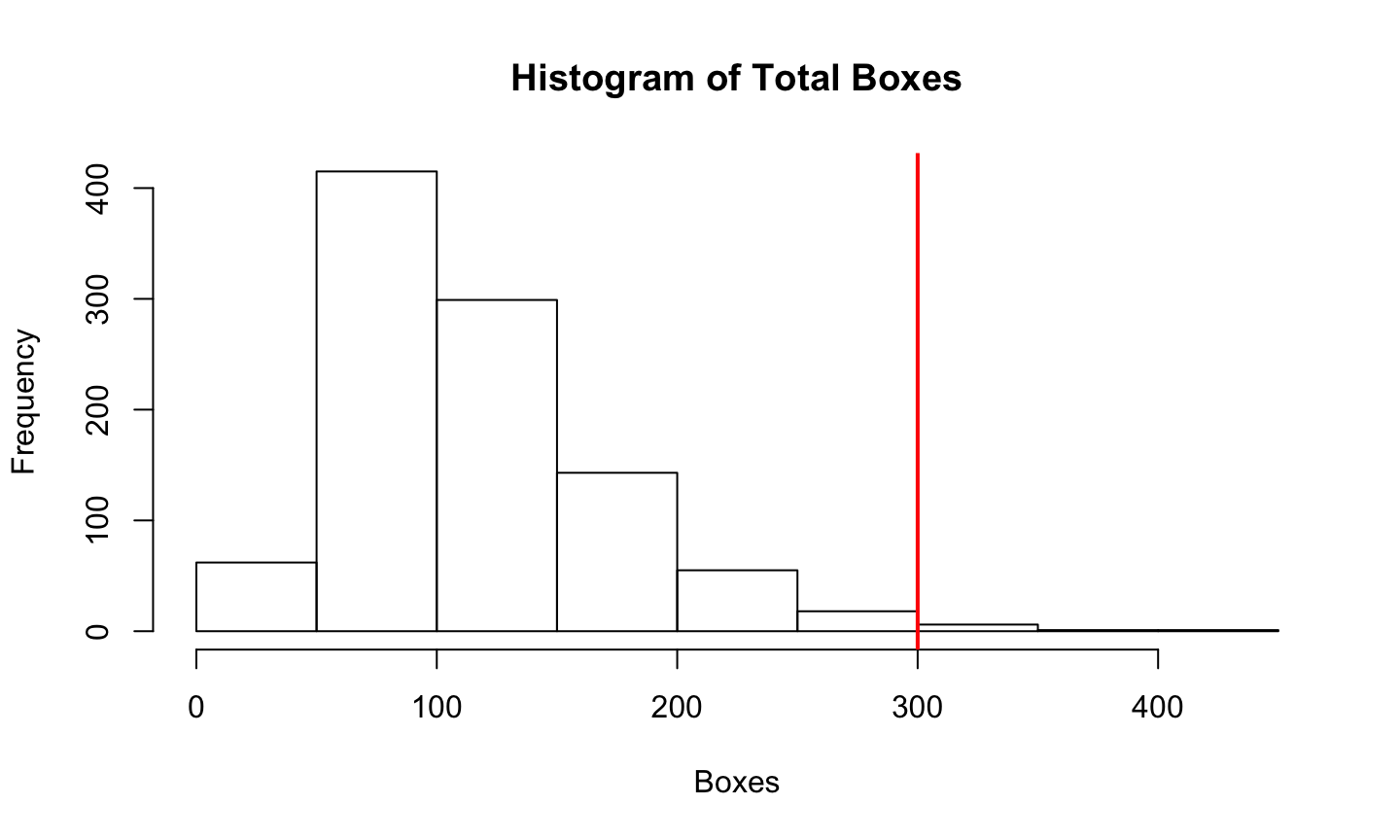## Plug-In and the Bootstrap

• The worst mistake one can make in statistics is to confuse the sample and the population or to confuse estimators and parameters
• That is, $$\hat{\theta}$$ is not $$\theta$$
• Plug-in principle seems to say the opposite
• Sometimes it is okay to just plug in an estimate for an unknown parameter
• In particular, okay to plug in a consistent estimator of the asymptotic variance of a parameter in forming asymptotic confidence intervals for that parameter
• So it is a terrible mistake to confuse a parameter of interest and an estimator for it, but it may not be a mistake to ignore the difference between a nuisance parameter and an estimator for it

## Plug-In and the Bootstrap

• The “bootstrap” is a cute name for a vast generalization of the plug-in principle
• The name comes from the cliche "pull oneself up by one’s bootstraps" which although it describes a literal impossibility actually means succeed by one’s own efforts
• In statistics, the hint of impossibility is part of the flavor
• Seems problematic, but it (usually) works

## Nonparametric Bootstrap

• The bootstrap comes in two flavors, parametric and nonparametric
• Theory of the nonparametric bootstrap is all above the level of this course, so we give a non-theoretical explanation
• The nonparametric bootstrap, considered non-theoretically, is just an analogy

## Nonparametric Bootstrap

World Real Bootstrap
true distribution $$F$$ $$\hat{F}_n$$
data $$X_1, \dots, X_n$$ IID $$F$$ $$X_1^*, \dots, X_n^*$$ IID $$\hat{F}_n$$
empirical distribution $$\hat{F}_n$$ $$F_n^*$$
parameter $$\theta = t(F)$$ $$\hat{\theta}_n = t(\hat{F}_n)$$
estimator $$\hat{\theta}_n = t(\hat{F}_n)$$ $$\theta_n^* = t(F_n^*)$$
error $$\hat{\theta}_n - \theta$$ $$\theta_n^* - \hat{\theta}_n$$
standardized error $$\frac{\hat{\theta}_n - \theta}{s(\hat{F}_n)}$$ $$\frac{\theta_n^* - \hat{\theta}_n}{s(F_n^*)}$$

Notation $$\theta = t(F)$$ means $$\theta$$ is some function of the true unknown distribution

## Nonparametric Bootstrap

• The notation $$X_1^*, \dots, X_n^*$$ IID $$\hat{F}_n$$ means $$X_1^*, \dots, X_n^*$$ are independent and identically distributed from the empirical distribution of the real data
• Sampling from the empirical distribution is just like sampling from a finite population, where the “population” is the real data $$X_1, \dots, X_n$$
• To be IID sampling must be with replacement
• $$X_1^*, \dots, X_n^*$$ are a sample with replacement from $$X_1, \dots, X_n$$
• Called resampling

## Nonparametric Bootstrap

• We want to know the sampling distribution of $$\hat{\theta}_n$$ or $$\hat{\theta}_n - \theta$$ or $$\frac{\hat{\theta}_n - \theta}{s(\hat{F}_n)}$$
• This sampling distribution depends on the true unknown distribution $$F$$ of the real data
• May be very difficult or impossible to calculate theoretically
• Even asymptotic approximation may be difficult, if the parameter $$\theta = t(F)$$ is a sufficiently complicated function of the true unknown $$F$$
• The statistical theory we have covered is quite amazing in what it does, but there is a lot it doesn’t do

## Nonparametric Bootstrap

• In the “bootstrap world” everything is known, $$\hat{F}_n$$ plays the role of the true unknown distribution and $$\hat{\theta}_n$$ plays the role of the true unknown parameter value
• The sampling distribution of $$\theta_n^*$$ or $$\theta_n^* - \hat{\theta}_n$$ or $$\frac{\theta_n^* - \hat{\theta}_n}{s(F_n^*)}$$ may still be difficult to calculate theoretically, but it can always be “calculated” by simulation.

## Nonparametric Bootstrap

• The bootstrap is large sample, approximate, asymptotic
• It is not an exact method
• The bootstrap analogy works when the empirical distribution $$\hat{F}_n$$ is close to the true unknown distribution $$F$$
• Usually the case when the sample size $$n$$ is large and not otherwise

## Bootstrap Percentile Intervals

• Simplest method of making confidence intervals for the unknown parameter is to take $$\alpha/2$$ and $$1 - \alpha/2$$ quantiles of the bootstrap distribution of the estimator $$\theta_n^*$$ as endpoints of the 100(1 − $$\alpha$$)% confidence interval
• Percentile method only makes sense when there is a symmetrizing transformation (some function of $$\hat{\theta}$$ has an approximately symmetric distribution with the center of symmetry being the true unknown parameter value $$\theta$$)
• The symmetrizing transformation does not have to be known, but it does have to exist

## Parametric Bootstrap

• The parametric bootstrap is just like the nonparametric bootstrap except for one difference in the analogy
• We use a parametric model $$F_{\hat{\theta}_n}$$ rather than the empirical distribution $$\hat{F}_n$$ as the analog of the true unknown distribution in the bootstrap world

## Parametric Bootstrap

World Real Bootstrap
parameter $$\theta$$ $$\hat{\theta}_n$$
true distribution $$F_{\theta}$$ $$F_{\hat{\theta}_n}$$
data $$X_1, \dots, X_n$$ IID $$F_{\theta}$$ $$X_1^*, \dots, X_n^*$$ IID $$F_{\hat{\theta}_n}$$
estimator $$\hat{\theta}_n = t(X_1, \dots, X_n)$$ $$\theta_n^* = t(X_1^*, \dots, X_n^*)$$
error $$\hat{\theta}_n - \theta$$ $$\theta_n^* - \hat{\theta}_n$$
standardized error $$\frac{\hat{\theta}_n - \theta}{s(X_1, \dots, X_n)}$$ $$\frac{\theta_n^* - \hat{\theta}_n}{s(X_1^*, \dots, X_n^*)}$$

## Parametric Bootstrap

• Simulation from the parametric model $$F_{\hat{\theta}_n}$$ not analogous to finite population sampling and does not resample the data like the nonparametric bootstrap does
• Instead we simulate the parametric model
• May be easy (when R has a function to provide such random simulations) or difficult

## Nonparametric versus Parametric

• The nonparametric bootstrap is nonparametric. That means it always does the right thing, except when it doesn’t. It doesn’t work when the sample size is too small or when the square root law doesn’t hold or when the data are not IID or when various technical issues arise that are beyond the scope of this course – the parameter is not a nice enough function of the true unknown distribution.
• The parametric bootstrap is parametric. That means it is always wrong when the model is wrong. On the other hand, when the parametric bootstrap does the right thing (when the statistical model is correct), it does a much better job at smaller sample sizes than the nonparametric bootstrap.

## Nonparametric versus Parametric

• When the parameter $$\theta$$ is defined in terms of the parametric statistical model and can only be estimated using the parametric model (by maximum likelihood perhaps), the statistical model is needs to be correct for the parameter estimate $$\hat{\theta}_n$$ to make sense
• Since we already need the statistical model to be correct, the parametric bootstrap is the logical choice

## Abnormal speed of light data

• Thanks to Rob Gould (UCLA Statistics) for the following example
• In 1882 Simon Newcomb performed an experiment to measure the speed of light
• Measured time it took for light to travel from Fort Myer on the west bank of the Potomac River to a fixed mirror at the foot of the Washington monument 3721 meters away
• In the units of the data, the currently accepted "true" speed of light is 33.02
• Does the data support the current accepted speed of 33.02?
speed <- c(28, -44, 29, 30, 26, 27, 22, 23, 33, 16, 24, 29, 24, 40 , 21, 31, 34, -2, 25, 19)
• To convert these units to time in the millionths of a second, multiply by $$10^{-3}$$ and add $$24.8$$

## Abnormal speed of light data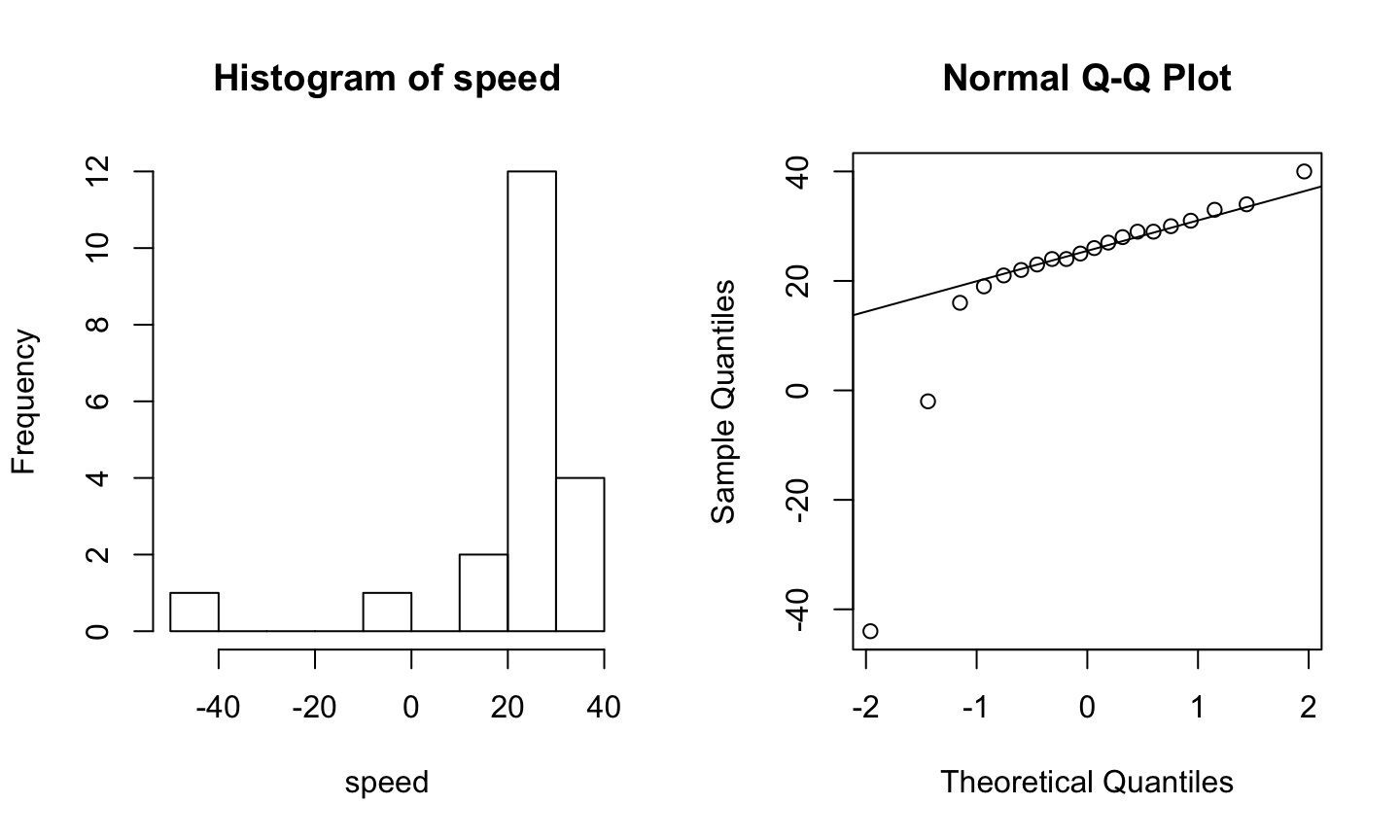## Abnormal speed of light data

• A $$t$$-test assumes the population of measurements is normally distributed
• With this small sample size and a severe departure from normality, we can’t be guaranteed a good approximation
• Instead, we can consider the bootstrap

## Abnormal speed of light data

1. State null and alternative hypotheses $H_0: \mu = 33.02 \text{ versus } H_a: \mu \ne 33.02$
2. Choose a significance level, in our case 0.05
3. Choose a test statistic, since we wish to estimate the mean speed we can use the sample average
4. Find the observed value of the test statistic
5. Calculate a p-value?
mean(speed)
##  21.75

## Abnormal speed of light data

• We now need a p-value, but we don't have the sampling distribution of our test statistic when the null hypothesis is true
• It is approximately normal, but that is a poor approximation here
• Instead we can perform a simulation under conditions in which we know the null hypothesis is true
• Use our data to represent the population, but first we shift it over so that the mean really is 33.02
newspeed <- speed - mean(speed) + 33.02
• Histogram of newspeed will have exactly the same shape as speed, but will be shifted

## Abnormal speed of light data

• Now we reach into our fake population and take out 20 observations at random, with replacement
• We take out 20 because that’s the size of our initial sample
• We calculate the average and save it, then repeat this process many, many times
• Now we have a sampling distribution with mean 33.02
• Can compare this to our observed sample average and obtain a p-value
n <- 1000
bstrap <- double(n)
for (i in 1:n){
newsample <- sample(newspeed, 20, replace=T)
bstrap[i] <- mean(newsample)
}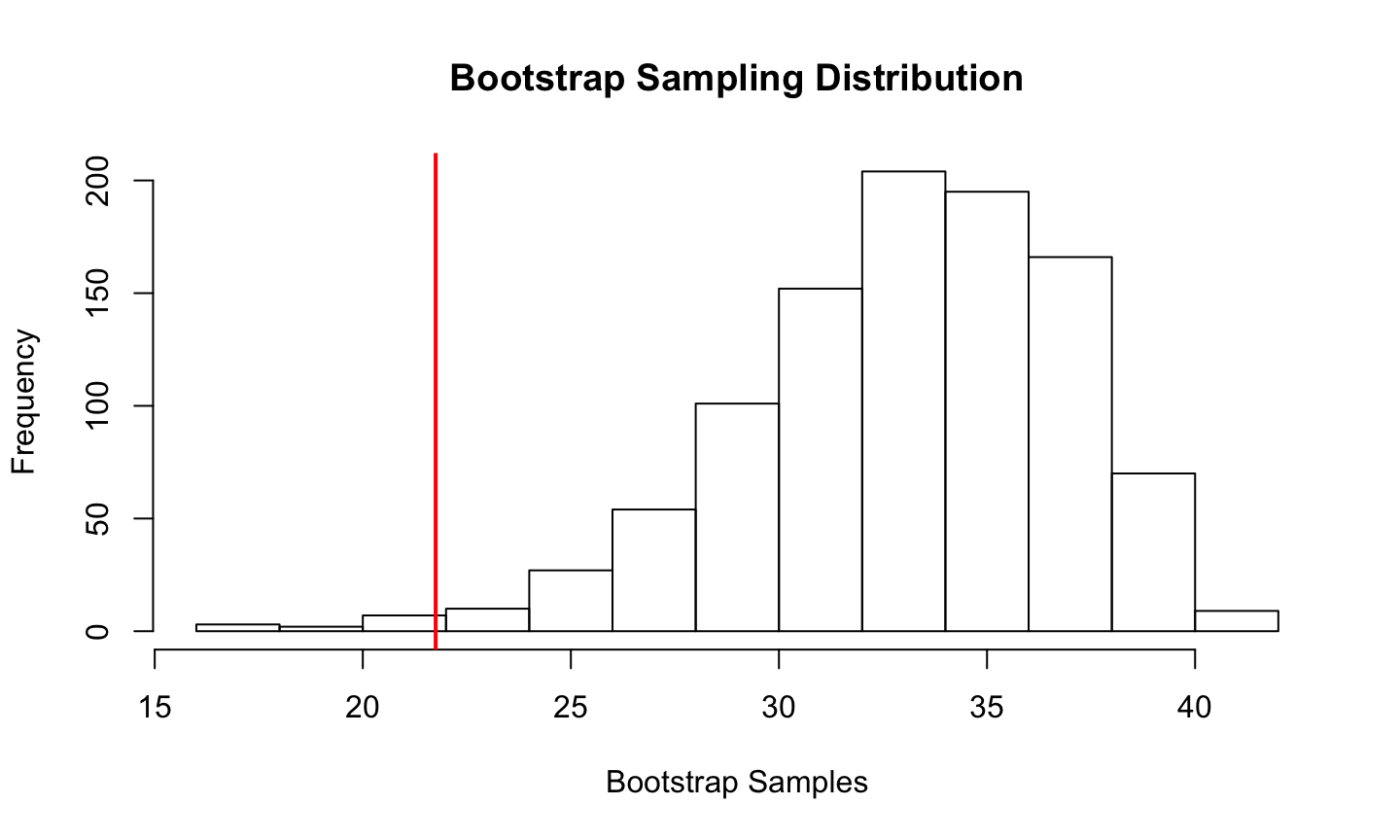• Doesn’t look normal, which means we did the right thing
• Not impossible for the sample average to be 21.75
• But it’s not all that common, either

## Abnormal speed of light data

• The p-value is the probability of getting something more extreme than what we observed
• Notice $$21.75$$ is $$33.02 - 21.75 = 11.27$$ units away from the null hypothesis
• So p-value is the probability of being more than 11.27 units away from 33.02
(sum(bstrap < 21.75) + sum(bstrap > 44.29))/1000
##  0.011
• Since our significance level is 5%, we reject $$H_0$$ and conclude that Newcomb’s measurements were not consistent with the currently accepted figure

## Example: Sleep study

• The two sample $$t$$-test checks for differences in means according to a known null distribution
• Similar to permutation tests
• Let's resample and generate the sampling distribution under the bootstrap assumption
bootstrap.resample <- function (object) sample (object, length(object), replace=TRUE)
diff.in.means <- function(df) {
mean(df[df$group==1,"extra"]) - mean(df[df$group==2,"extra"])
}
resample.diffs <- replicate(2000, diff.in.means(sleep[bootstrap.resample(1:nrow(sleep)),]))

## Example: Sleep study

hist(resample.diffs, main="Bootstrap Sampling Distribution")
abline(v=diff.in.means(sleep), col=2, lwd=3)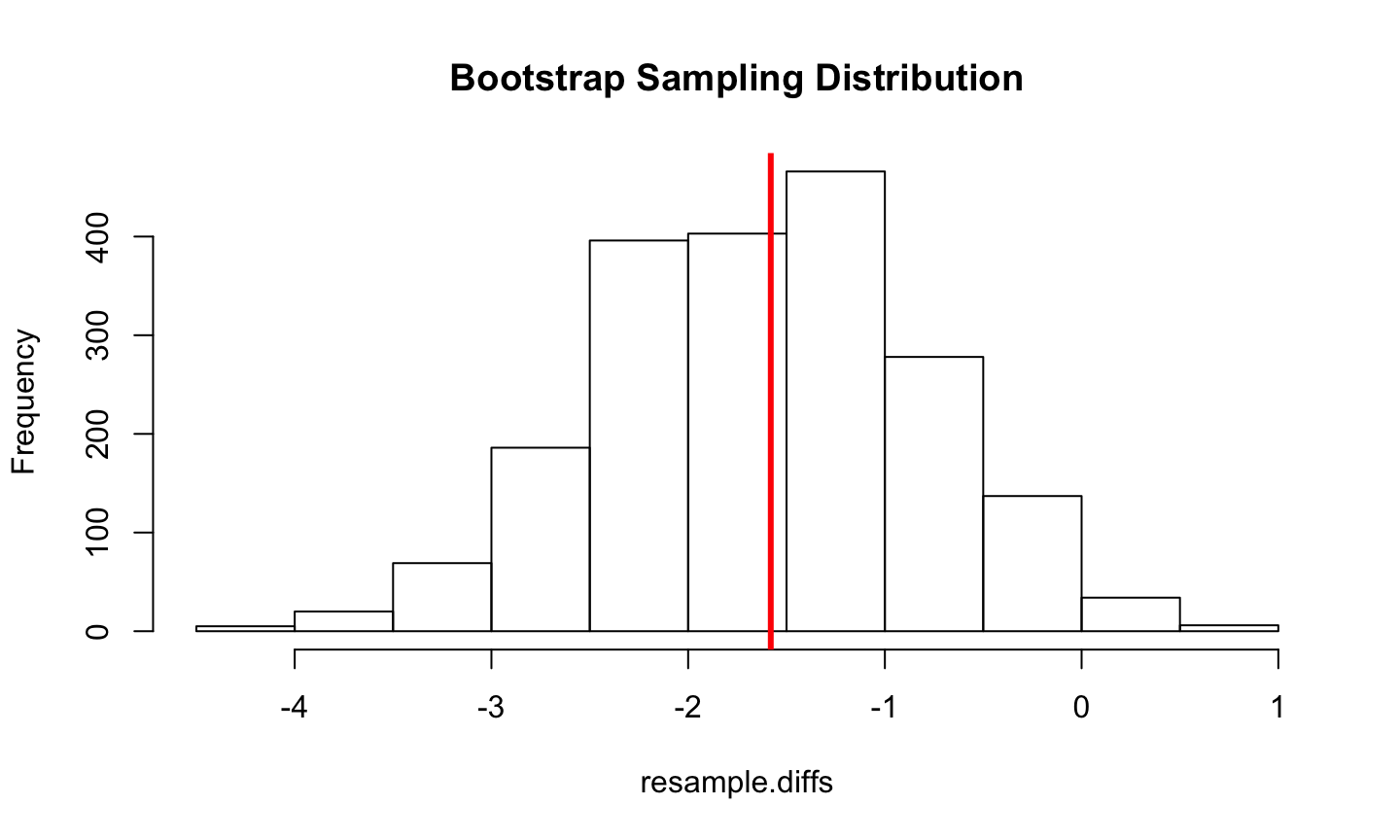## Bootstrapping functions

• R has numerous built in bootstrapping functions, too many to mention, see boot library
• Example of the function boot
• Bootstrap of the ratio of means using the city data included in the boot package
library(boot)
data(city)
ratio <- function(d, w) sum(d$x * w)/sum(d$u * w)
results <- boot(city, ratio, R=1000, stype="w")

## Bootstrapping functions

results
##
## ORDINARY NONPARAMETRIC BOOTSTRAP
##
##
## Call:
## boot(data = city, statistic = ratio, R = 1000, stype = "w")
##
##
## Bootstrap Statistics :
##     original     bias    std. error
## t1* 1.520313 0.03619229   0.2300674

## Bootstrapping functions

boot.ci(results, type="bca")
## BOOTSTRAP CONFIDENCE INTERVAL CALCULATIONS
## Based on 1000 bootstrap replicates
##
## CALL :
## boot.ci(boot.out = results, type = "bca")
##
## Intervals :
## Level       BCa
## 95%   ( 1.275,  2.183 )
## Calculations and Intervals on Original Scale

## Bootstrapping a single statistic

• Can use the bootstrap to generate a 95% confidence interval for R-squared
• Linear regression of miles per gallon (mpg) on car weight (wt) and displacement (disp)
• Data source is mtcars
• The bootstrapped confidence interval is based on 1000 replications
rsq <- function(formula, data, indices) {
d <- data[indices,]
fit <- lm(formula, data=d)
return(summary(fit)\$r.square)
}
results <- boot(data=mtcars, statistic=rsq,
R=1000, formula=mpg~wt+disp)

## Bootstrapping a single statistic

results
##
## ORDINARY NONPARAMETRIC BOOTSTRAP
##
##
## Call:
## boot(data = mtcars, statistic = rsq, R = 1000, formula = mpg ~
##     wt + disp)
##
##
## Bootstrap Statistics :
##      original     bias    std. error
## t1* 0.7809306 0.00958034  0.05058801

## Bootstrapping a single statistic

boot.ci(results, type="bca")
## BOOTSTRAP CONFIDENCE INTERVAL CALCULATIONS
## Based on 1000 bootstrap replicates
##
## CALL :
## boot.ci(boot.out = results, type = "bca")
##
## Intervals :
## Level       BCa
## 95%   ( 0.6424,  0.8557 )
## Calculations and Intervals on Original Scale
## Some BCa intervals may be unstable

## Bootstrapping a single statistic

plot(results)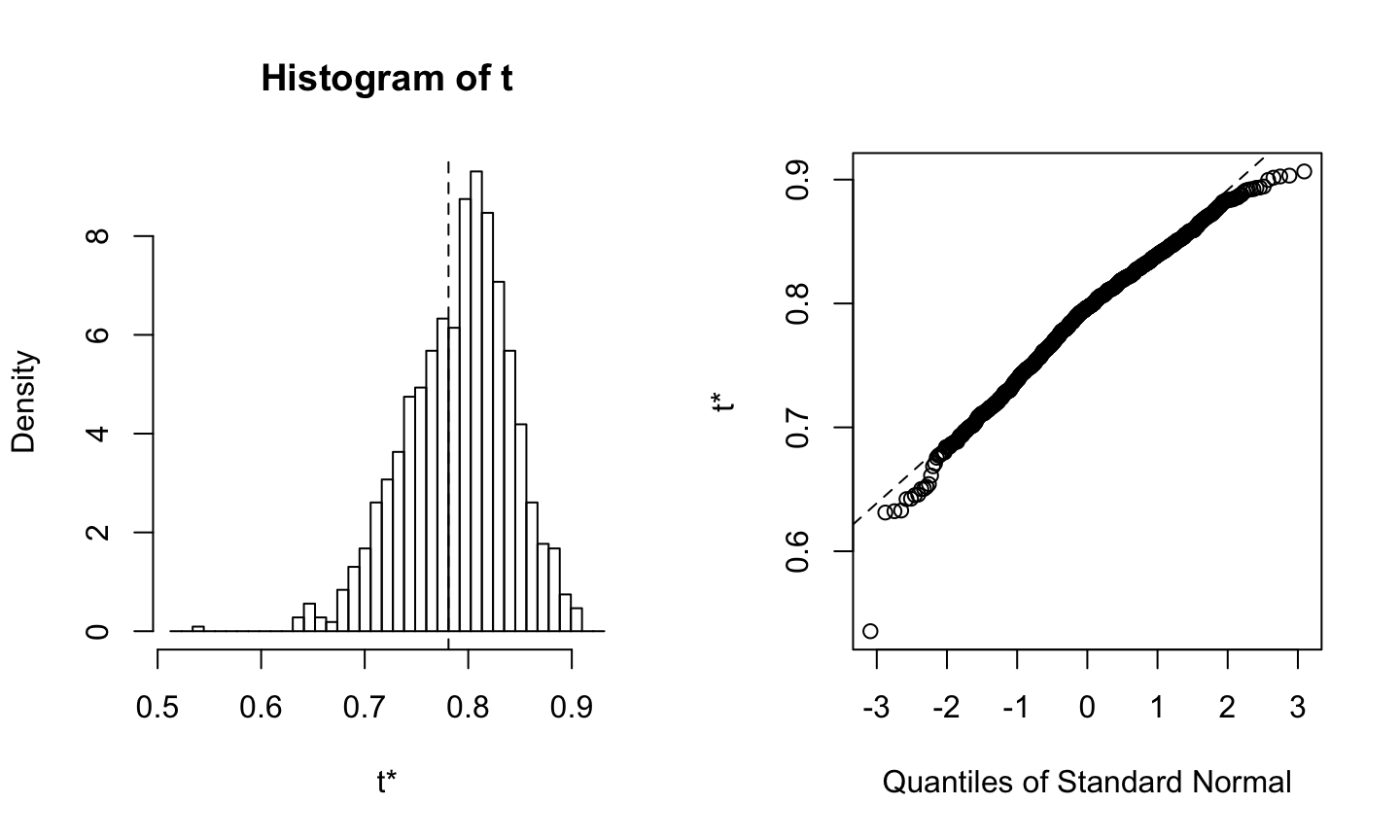## Summary

• Bootstrapping provides a nonparametric approach to statistical inference when distributional assumptions may not be met
• Enables calculation of standard errors and conﬁdence intervals in a variety of situations, e.g. medians, correlation coefficients, regression parameters, …
• Hypothesis tests are a little more challenging
• The bootstrap is large sample, approximate, and asymptotic!
• Works when the empirical distribution $$\hat{F}_n$$ is close to the true unknown distribution $$F$$
• Usually the case when the sample size $$n$$ is large and not otherwise, no method can save bad data!